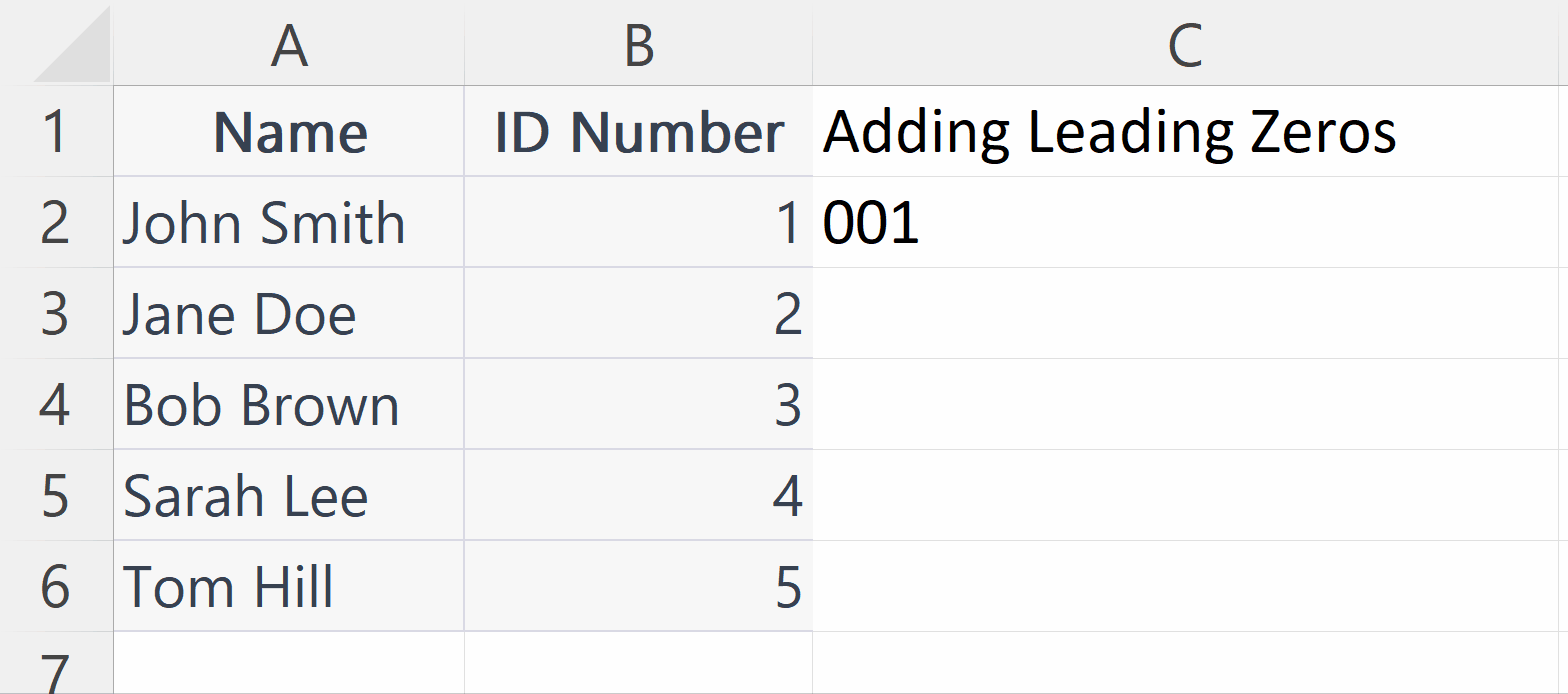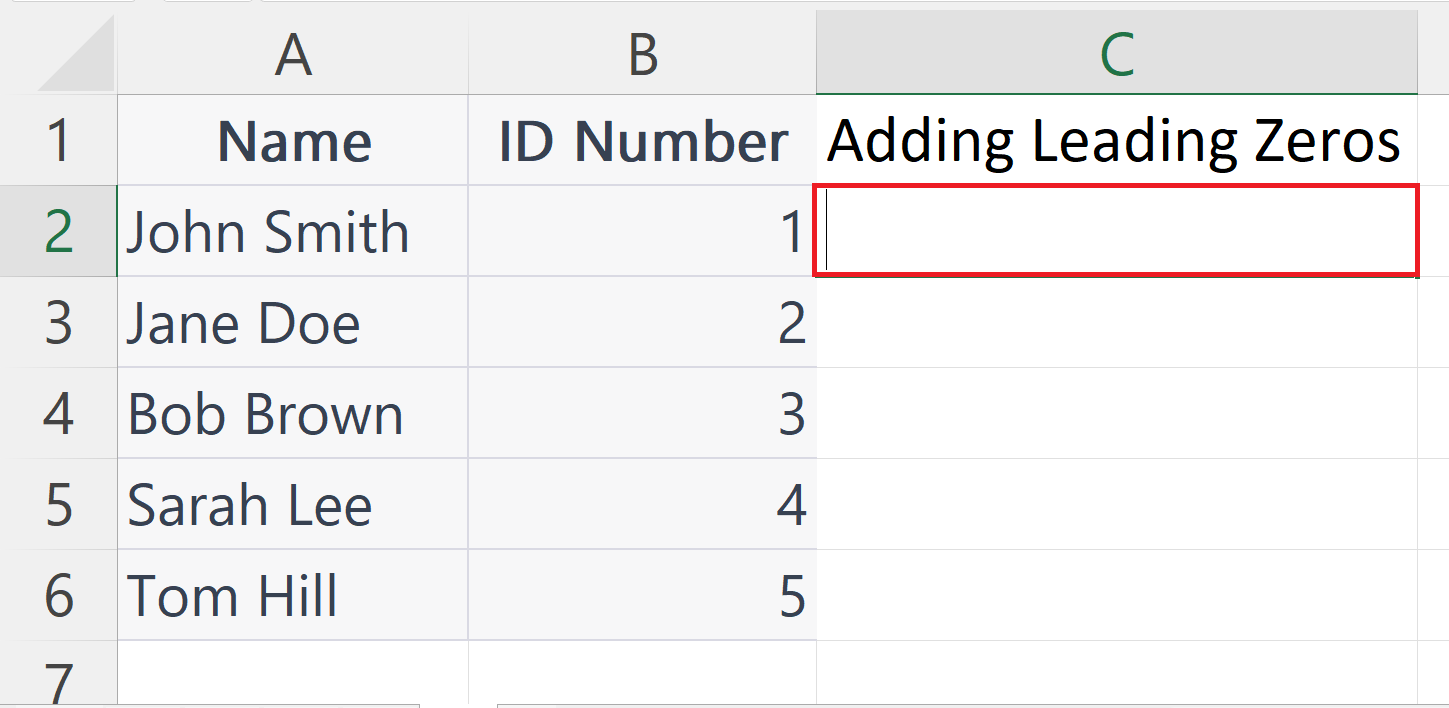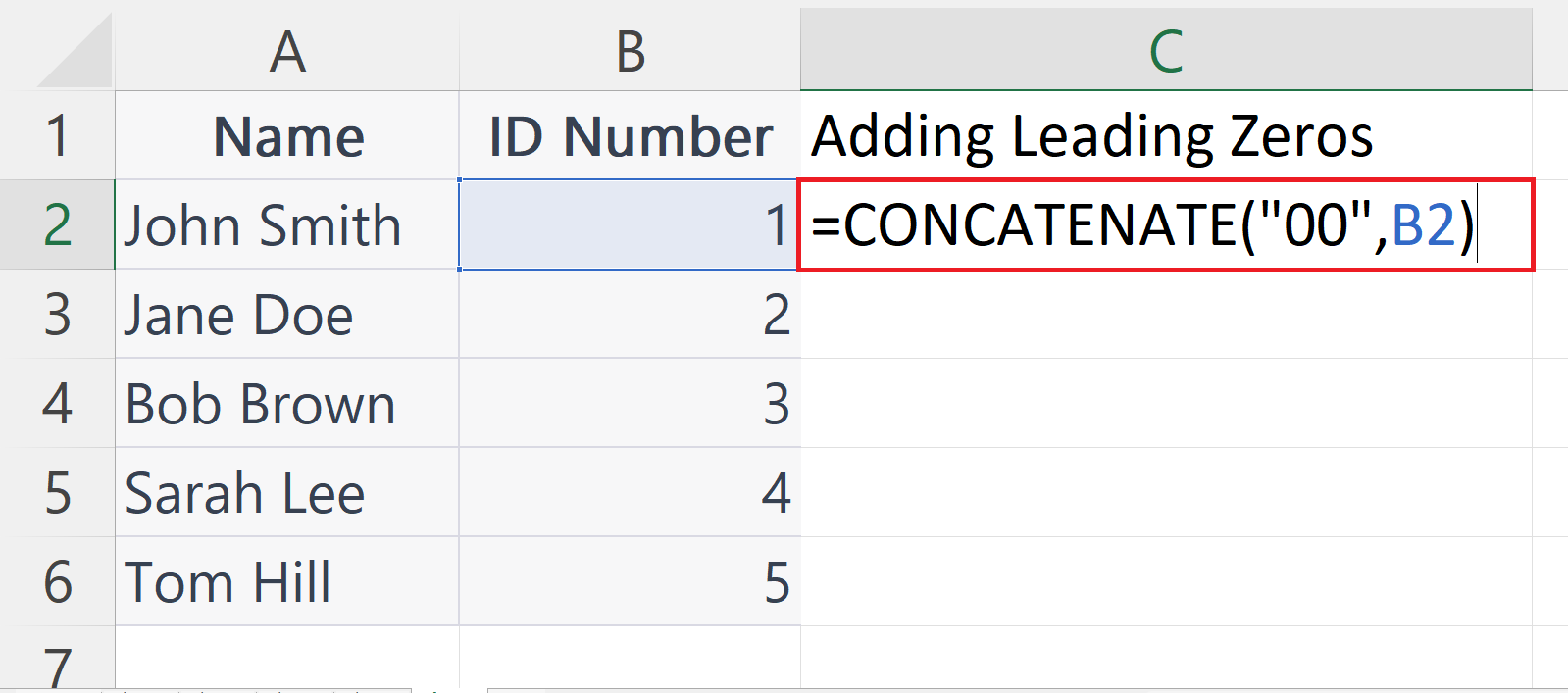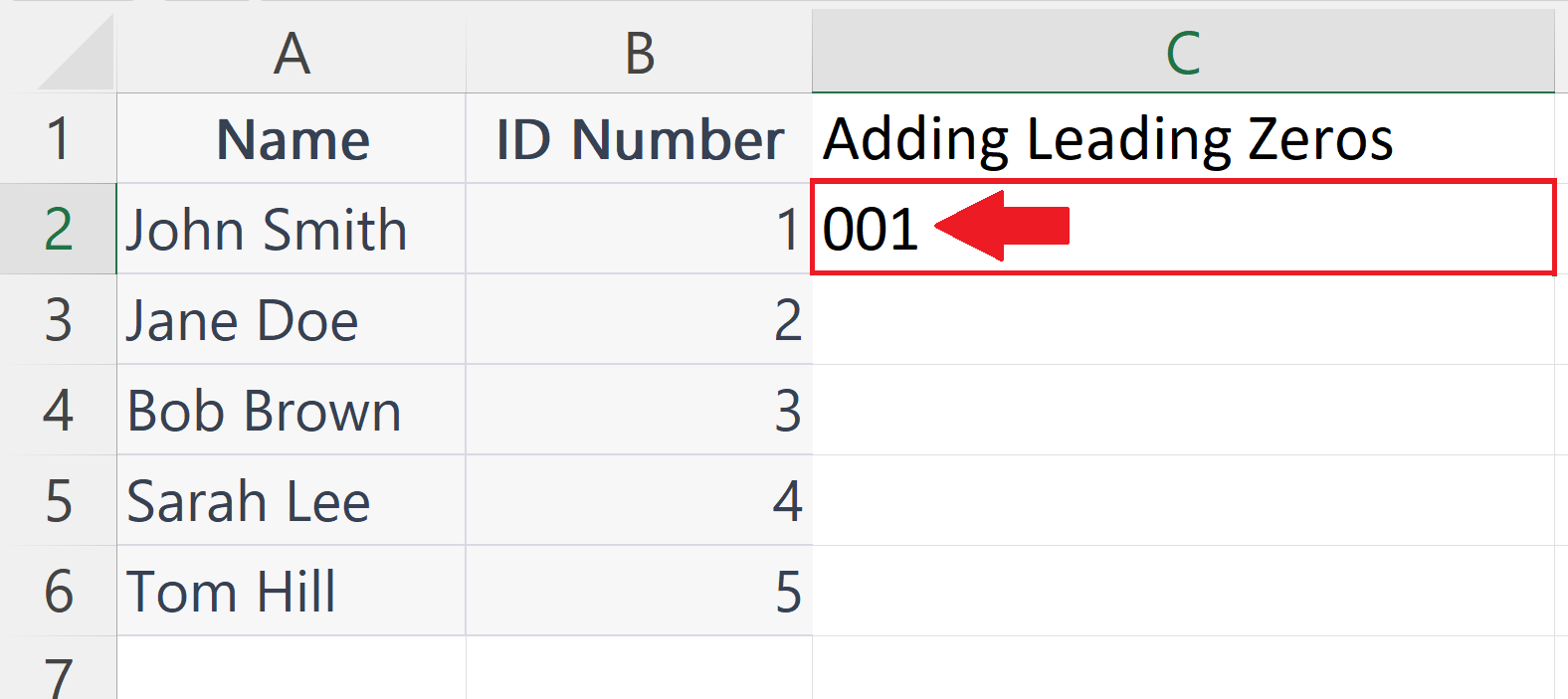# How to add leading zeros in Microsoft Excel using CONCATENATE function

In this tutorial we will learn how to add leading zeros in Microsoft Excel using the CONCATENATE function. Adding leading zeros to a number in Excel is a straightforward process. One way to accomplish this is by utilizing the CONCATENATE function. This function is a versatile tool that enables the merging of text or numerical values from various cells into a single cell. It proves particularly handy in scenarios where data needs to be merged from diverse sources, thereby saving time and effort.

The ID numbers in this dataset have two leading zeros in their original format. To preserve this format, we can add two leading zeros to each number using the CONCATENATE function.In Microsoft Excel, leading zeros refer to the digits that appear before the first significant digit in a cell. These zeros are often used to maintain consistent formatting and ensure that numbers are displayed correctly. For example, if you want to display a set of account numbers that all have the same number of digits, you may need to add leading zeros to some of the numbers to make them the same length. In Microsoft Excel, it’s a common occurrence that leading zeros disappear when you insert a number. This is because, in most cases, leading zeros do not hold any significant value.

### Step 1 – Select a Blank Cell– Select a blank cell where you want to print the ID number with leading zeros.

### Step 2 – Place an Equals Sign– Place an Equals sign ( = ) in the targeted blank cell.

### Step 3 – Use the CONCATENATE Function– The syntax for CONCATENATE function to add 2 leading zeros will be:
CONCATENATE(“00”,A2)
– The first argument i.e. “00”  are the leading zeros to be added.
– The second argument i.e. A2 is the address of the cell containing the ID number in which leading zeros are to be added.

### Step 4 – Press the Enter Key